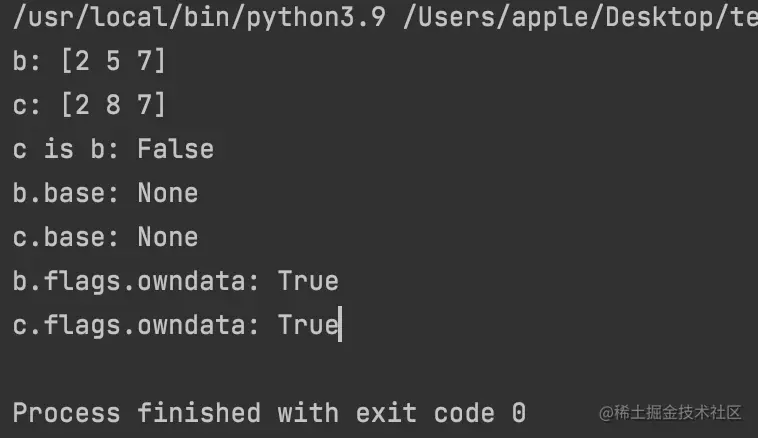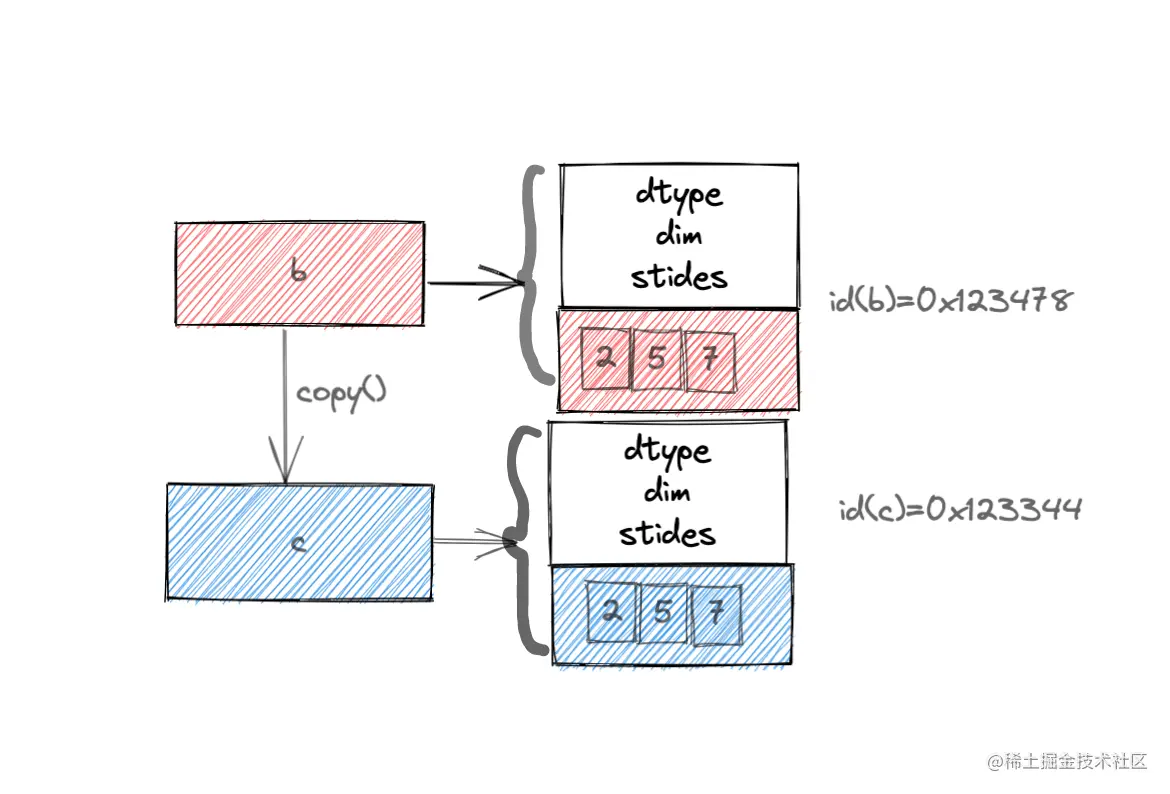﻿ Python numpy视图与副本_python_脚本之家
python# Python numpy视图与副本`metdata `：存放数组类型dtype、数组维度ndim、维度数量shape、维间距strides等
`raw bata`：存放原始数据data

`metdata `中包含着关于数组相关信息，可以帮助我们在数组`ndarray`中快速索引和解释指定的数据

## 1. 简单讲解

ndarray.flags : 查看数组存储策略、读写权限、对象等

• `C_CONTIGUOUS (C)` 行优先存储
• `F_CONTIGUOUS` 列优先存储
• `OWNDATA `数据所有者
• `WRITEABLE `编写权限
• `ALIGNED `数据元素与硬件指针对齐
• `WRITEBACKIFCOPY` 数组是其他数组的副本
• `UPDATEIFCOPY `已弃用

• `ndarray.base `: 查看数组中的元素是否来自其他数组
• `ndarray.nbytes`: 查看数组中数据占用的字节数
• `getsizeof(item)`: 查看数组占用的内存空点

```>>> import numpy as np
>>> a = np.array([1,2,3,4])
>>> print(a[1:3])
[2 3]
>>> print(a[[1,2]])
[2 3]
>>>```

```>>> print(id(a[1:3]))
2247482965008
>>> print(id(a[[1,2]]))
2247482964928```

```>>> print(a.flags.owndata)
True
>>> print(a[1:3].flags.owndata)
False
>>> print(a[[1,2]].flags.owndata)
True```

a[[1,2]] 是数据所有者，数据来源本身（None）

```>>> print(a[[1,2]].base)
None
>>> print(a[1:3].base)
[1 2 3 4]```

## 2. 视图

### 视图概念

No copy at All。 Simple assignments make no copy of objects or their data.

### 视图应用

• 当对原始数组进行引用时
• 当自身无数据，与原数组共享数据时
```>>> import numpy as np
>>> a = np.array([1,2,3,4])
>>> b = a
>>> b is a
True
>>> id(a)
2247207679680
>>> id(b)
2247207679680
>>>```

`numpy `模块诸如索引、切片、函数`view()`,`reshape()`等返回视图结果

```>>> arr = np.arange(10)
>>> arr_view = arr.view()
>>> arr.shape = (2,5)
>>> arr_reshape = arr.reshape(5,2)
# ndarray.base 属性
>>> print(arr.base)
None
>>> print(arr_view.base)
[[0 1 2 3 4]
[5 6 7 8 9]]
>>> print(arr_reshape.base)
[[0 1 2 3 4]
[5 6 7 8 9]]
# ndarray.flags.owndata 属性
>>> print(arr.flags.owndata)
True
>>> print(arr_view.flags.owndata)
False
>>> print(arr_reshape.flags.owndata)
False
>>>```## 3. 副本

### 副本概念

Deep Copy The copy method makes a complete copy of the array and its data

### 副本应用

• 当进行切片操作时
• 当需要与原始数组数据独立时

```b = np.array([2,5,7])

c = b.copy()

c = 8

print("b:",b)
print("c:",c)

print("c is b:",c is b)

# 查看 ndarray.base 属性å
print("b.base:",b.base)
print("c.base:",c.base)

# 查看 ndarray.flags.owndata
print("b.flags.owndata:",b.flags.owndata)
print("c.flags.owndata:",c.flags.owndata)```• 视图，相当于浅拷贝，与原数组共享数据。
• 副本，相当于深拷贝，与原数组数据相互独立